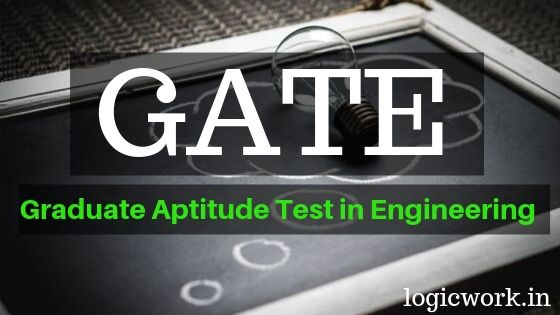# Gate Syllabus for Electrical Engineering (EE) pdf

Download Gate Syllabus for Electrical Engineering (EE) pdf – As a GATE aspirant gate syllabus is the first step to clear the exam.

### GATE 2020 Syllabus and Paper Pattern

 GATE Paper (Code) Electrical Engineering (EE) Total Number of Questions 65 Total Marks 100 Time Duration of Exam 3 Hours Marks Distribution 15 Marks from General Aptitude15 Marks from Engineering Mathematics70 Marks from Core Subjects of Paper

#### 1. General Aptitude

Verbal Ability: English grammar, sentence completion, verbal analogies, word groups, instructions, critical reasoning and verbal deduction.
Numerical Ability: Numerical computation, numerical estimation, numerical reasoning and data interpretation.

#### 2. Engineering Mathematics

Linear Algebra: Matrix Algebra, Systems of linear equations, Eigenvalues, Eigenvectors.
Calculus: Mean value theorems, Theorems of integral calculus, Evaluation of definite and improper integrals, Partial Derivatives, Maxima and minima, Multiple integrals, Fourier series, Vector identities, Directional derivatives, Line integral, Surface integral, Volume integral, Stokes’s theorem, Gauss’s theorem, Green’s theorem.

Differential Equations: First order equations (linear and nonlinear), Higher order linear differential equations with constant coefficients, Method of variation of parameters, Cauchy’s equation, Euler’s equation, Initial and boundary value problems, Partial Differential Equations, Method of separation of variables.

Complex variables: Analytic functions, Cauchy’s integral theorem, Cauchy’s integral formula, Taylor series, Laurent series, Residue theorem, Solution integrals.

Probability and Statistics: Sampling theorems, Conditional probability, Mean, Median, Mode, Standard Deviation, Random variables, Discrete and Continuous distributions, Poisson distribution, Normal distribution, Binomial distribution, Correlation analysis, Regression analysis.
Numerical Methods: Solutions of nonlinear algebraic equations, Single and Multi-step methods for differential equations.

Transform Theory: Fourier Transform, Laplace Transform, z-Transform.

#### 3. Electric Circuits

Network graph, KCL, KVL, Node and Mesh analysis, Transient response of dc and ac networks, Sinusoidal steadystate analysis, Resonance, Passive filters, Ideal current and voltage sources, Thevenin’s theorem, Norton’s theorem, Superposition theorem, Maximum power transfer theorem, Two-port networks, Three phase circuits, Power and power factor in ac circuits.

#### 4. Electromagnetic Fields

Coulomb’s Law, Electric Field Intensity, Electric Flux Density, Gauss’s Law, Divergence, Electric field and potential due to point, line, plane and spherical charge distributions, Effect of dielectric medium, Capacitance of simple configurations, Biot-Savart’s law, Ampere’s law, Curl, Faraday’s law, Lorentz force, Inductance, Magnetomotive force, Reluctance, Magnetic circuits,Self and Mutual inductance of simple configurations.

#### 5. Signals and Systems

Representation of continuous and discrete-time signals, Shifting and scaling operations, Linear Time Invariant and Causal systems, Fourier series representation of continuous periodic signals, Sampling theorem, Applications of Fourier Transform, Laplace Transform and z-Transform.

#### 6. Electrical Machines

Single phase transformer: equivalent circuit, phasor diagram, open circuit and short circuit tests, regulation and efficiency;

Three phase transformers: connections, parallel operation; Auto-transformer, Electromechanical energy conversion principles, DC machines: separately excited, series and shunt, motoring and generating mode of operation and their characteristics, starting and speed control of dc motors;

Three phase induction motors: principle of operation, types, performance, torque-speed characteristics, no-load and blocked rotor tests, equivalent circuit, starting and speed control; Operating principle of single phase induction motors;

Synchronous machines: cylindrical and salient pole machines, performance, regulation and parallel operation of generators, starting of synchronous motor, characteristics; Types of losses and efficiency calculations of electric machines.

#### 7. Power Systems

Power generation concepts, ac and dc transmission concepts, Models and performance of transmission lines and cables, Series and shunt compensation, Electric field distribution and insulators, Distribution systems, Per-unit quantities, Bus admittance matrix, Gauss-Seidel and Newton-Raphson load flow methods, Voltage and Frequency control, Power factor correction, Symmetrical components, Symmetrical and unsymmetrical fault analysis, Principles of over-current, differential and distance protection; Circuit breakers, System stability concepts, Equal area criterion.

#### 8. Control Systems

Mathematical modeling and representation of systems, Feedback principle, transfer function, Block diagrams and Signal flow graphs, Transient and Steady-state analysis of linear time invariant systems, Routh-Hurwitz and Nyquist criteria, Bode plots, Root loci, Stability analysis, Lag, Lead and Lead-Lag compensators; P, PI and PID controllers; State space model, State transition matrix.

#### 9. Electrical and Electronic Measurements

Bridges and Potentiometers, Measurement of voltage, current, power, energy and power factor; Instrument transformers, Digital voltmeters and multimeters, Phase, Time and Frequency measurement; Oscilloscopes, Error analysis.

#### 10. Analog and Digital Electronics

Characteristics of diodes, BJT, MOSFET; Simple diode circuits: clipping, clamping, rectifiers; Amplifiers: Biasing, Equivalent circuit and Frequency response; Oscillators and Feedback amplifiers; Operational amplifiers: Characteristics and applications;

Simple active filters, VCOs and Timers, Combinational and Sequential logic circuits, Multiplexer, Demultiplexer, Schmitt trigger, Sample and hold circuits, A/D and D/A converters, 8085Microprocessor: Architecture, Programming and Interfacing.

#### 11. Power Electronics

Characteristics of semiconductor power devices: Diode, Thyristor, Triac, GTO, MOSFET, IGBT; DC to DC conversion: Buck, Boost and Buck-Boost converters; Single and three phase configuration of uncontrolled rectifiers, Line commutated thyristor based converters, Bidirectional ac to dc voltage source converters, Issues of line current harmonics, Power factor, Distortion factor of ac to dc converters, Single phase and three phase inverters, Sinusoidal pulse width modulation.